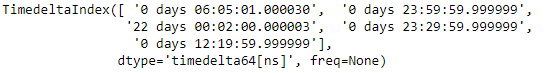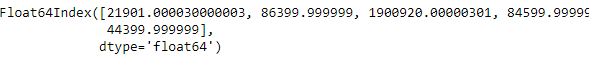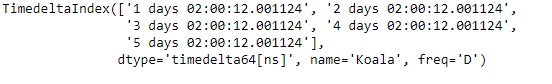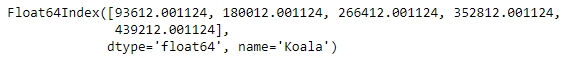# Python | Pandas TimedeltaIndex.total_seconds()

Python is a great language for doing data analysis, primarily because of the fantastic ecosystem of data-centric python packages. Pandas is one of those packages and makes importing and analyzing data much easier.

Pandas` TimedeltaIndex.total_seconds()` function is used to find the total duration of each element expressed in seconds. It returns an index object.

Syntax : TimedeltaIndex.total_seconds()

Parameters : None

Return : index

Example #1: Use `TimedeltaIndex.total_seconds()` function to find out the total time duration of each element of the given TimedeltaIndex object expressed in seconds.

 `# importing pandas as pd ` `import` `pandas as pd ` ` `  `# Create the TimedeltaIndex object ` `tidx ``=` `pd.TimedeltaIndex(data ``=``[``'06:05:01.000030'``, ``'+23:59:59.999999'``, ` `                        ``'22 day 2 min 3us 10ns'``, ``'+23:29:59.999999'``,  ` `                        ``'+12:19:59.999999'``]) ` ` `  `# Print the TimedeltaIndex object ` `print``(tidx) `

Output :Now we will use the `TimedeltaIndex.total_seconds()` function to find the total seconds for each element of the tidx object.

 `# time duration of each element expressed in seconds ` `tidx.total_seconds() `

Output :As we can see in the output, the `TimedeltaIndex.total_seconds()` function has returned an index object containing the time duration for each element of the TimedeltaIndex object expressed in seconds.

Example #2: Use `TimedeltaIndex.total_seconds()` function to find out the total time duration of each element of the given TimedeltaIndex object expressed in seconds.

 `# importing pandas as pd ` `import` `pandas as pd ` ` `  `# Create the TimedeltaIndex object ` `tidx ``=` `pd.TimedeltaIndex(start ``=``'1 days 02:00:12.001124'``, ` `                        ``periods ``=` `5``, freq ``=``'D'``, name ``=``'Koala'``) ` ` `  `# Print the TimedeltaIndex object ` `print``(tidx) `

Output :Now we will use the `TimedeltaIndex.total_seconds()` function to find the total seconds for each element of the tidx object.

 `# time duration of each element expressed in seconds ` `tidx.total_seconds() `

Output :As we can see in the output, the `TimedeltaIndex.total_seconds()` function has returned an index object containing the time duration for each element of the TimedeltaIndex object expressed in seconds.

My Personal Notes arrow_drop_upCheck out this Author's contributed articles.

If you like GeeksforGeeks and would like to contribute, you can also write an article using contribute.geeksforgeeks.org or mail your article to contribute@geeksforgeeks.org. See your article appearing on the GeeksforGeeks main page and help other Geeks.

Please Improve this article if you find anything incorrect by clicking on the "Improve Article" button below.

Article Tags :

Be the First to upvote.

Please write to us at contribute@geeksforgeeks.org to report any issue with the above content.